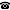# 2011 seminar talk: Topologically invariant \sigma-ideals on Polish spaces

Talk held by Taras Banakh (Lviv State University, Ukraine) at the KGRC seminar on 2011-06-22.

### Abstract

We shall discuss the structure of topologically invariant $$\sigma$$-ideals with Borel base on homogeneous Polish spaces. An ideal $$\mathcal I$$ of subsets of a topological space $$X$$ is called topologically invariant if for each set $$A\in\mathcal I$$ and each homeomorphism $$h:X\to X$$ we get $$h(A)\in\mathcal I$$.

During the lecture I plan to cover the following topics:

(1) classification of topologically invariant $$\sigma$$-ideals on topologically homogeneous zero-dimensional Polish spaces;

(2) extremal (i.e., maximal, largest, smallest) topologically invariant $$\sigma$$-ideals on some "nice" Polish spaces;

(3) cardinal characteristics of topologically invariant $$\sigma$$-ideals on some "nice" Polish spaces and their interplay with the cardinal characteristics of the ideal $$\mathcal M$$ of meager sets.

Kurt Gödel Research Center for Mathematical Logic. Währinger Straße 25, 1090 Wien, Austria.+43-1-4277-50501. Last updated: 2010-12-16, 04:37.